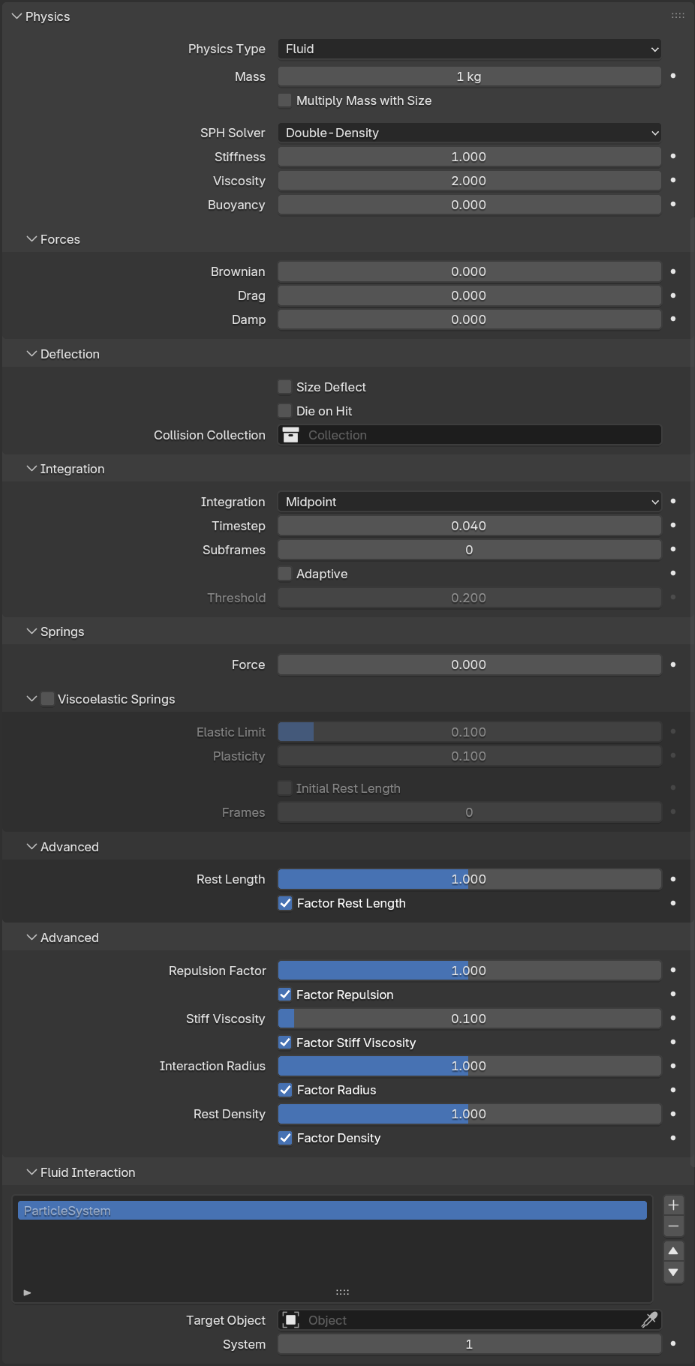# 流体¶

Reference

Panel: Particle System ‣ Physics 流体Fluid Physics settings.

Fluid particles are similar to Newtonian ones but this time particles are influenced by internal forces like pressure, surface tension, viscosity, springs, etc. From liquids to slime, goo to sand and wispy smoke the number of possible use cases is endless.

Blender particle fluids use the SPH techniques to solve the particles fluid equations. Smoothed-particle hydrodynamics (SPH) is a computational method used for simulating fluid flows. It has been used in many fields of research, including astrophysics, ballistics, vulcanology, and oceanography. It is a mesh-free Lagrangian method (where the coordinates move with the fluid), and the resolution of the method can easily be adjusted with respect to variables such as the density.

## Options¶

Fluid physics share options with Newtonian Physics. These are covered on that page.

### Fluid Properties¶

Stiffness
How incompressible the fluid is.
Viscosity
Linear viscosity. Use lower viscosity for thicker fluids.
Buoyancy
Artificial buoyancy force in negative gravity direction based on pressure differences inside the fluid.

Repulsion Factor
How strongly the fluid tries to keep from clustering (factor of stiffness). Checkbox sets repulsion as a factor of stiffness.
Stiff Viscosity
Creates viscosity for expanding fluid. Checkbox sets this to be a factor of normal viscosity.
Fluid's interaction radius. Checkbox sets this to be a factor of 4 × particle size.
Rest Density
Density of fluid when at rest. Checkbox sets this to be a factor of default density.

### Springs¶

Force
Spring force.
Rest Length
Rest length of springs. Factor of particle radius. Checkbox sets this to be a factor of 2 × particle size.
Viscoelastic Springs
Use viscoelastic springs instead of Hooke's springs.
Elastic Limit
How much the spring has to be stretched/compressed in order to change its rest length.
Plasticity
How much the spring rest length can change after the elastic limit is crossed.
Initial Rest Length
Use initial length as spring rest length instead of 2 × particle size.
Frames
Create springs for this number of frames since particle's birth (0 is always).